2021年09月06日

## 简介

Pytorch 由 Facebook 人工智能研究院于 2017 年推出，具有强大的 GPU 加速张量计算功能，并且能够自动进行微分计算，从而可以使用基于梯度的方法对模型参数进行优化。

## 什么是pytorch

Pytorch详细介绍(上)Pytorch是一个很容易学习、使用和部署的深度学习库。PyTorch同时提供了更高性能的C++ API（libtorch）。Pytorch使用Tensor作为核心数据结构，Tensor（张量）是神经网络世界的Numpy，其类似与Numpy数组，但是又不同于Numpy数组。为了提高Tensor的计算速度，有大量的硬件和软件为了Tensor运算进行支持和优化。总得来说，Tensor具有如下特点：

1. 具有类似Numpy的数据，Tensor可以与Numpy共享内存；
2. 可以指定计算设备，例如，设定Tensor是在CPU上计算，还是在GPU上计算；
3. Tensor可微分。
4. 通过访问张量的device属性可以获取张量所在的设备

PyTorch工作流以及与每个步骤相关联的重要模块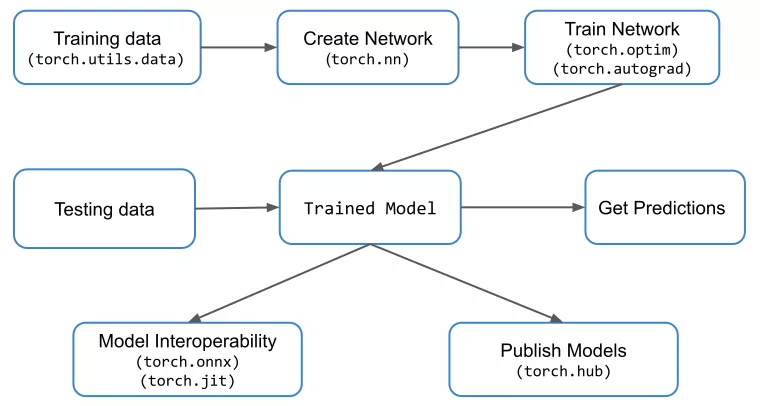[源码解析] PyTorch 分布式(8) ——– DistributedDataParallel 之 论文篇

1. PyTorch organizes values into Tensors which are generic n-dimensional arrays with a rich set of data manipulating operations.
2. A Module defines a transform from input val- ues to output values, and its behavior during the forward pass is specified by its forward member function. A Module can contain Tensors as parameters. For example, a Linear Module contains a weight parameter and a bias parameter, whose forward function generates the output by multiplying the input with the weight and adding the bias. An application composes its own Module by stitching together native Modules (e.g., linear, convolution, etc.) and Functions (e.g., relu, pool, etc.) in the custom forward function.
3. A typical training iteration contains a forward pass to generate losses using inputs and labels, a backward pass to compute gradients for parameters, and an optimizer step to update parameters using gradients. More specifically, during the forward pass, PyTorch builds an autograd graph to record actions performed. Then, in the backward pass, it uses the autograd graph to conduct backpropagation to generate gradients. Finally, the optimizer applies the gradients to update parameters. The training process repeats these three steps until the model converges.

## pytorch基础

### 张量

# 创建的张量会存储在内存中并使用 CPU 进行计算
>>> array = [[1.0, 3.8, 2.1], [8.6, 4.0, 2.4]]
>>> torch.tensor(array)
tensor([[1.0000, 3.8000, 2.1000],
[8.6000, 4.0000, 2.4000]])
# 在 GPU 中创建张量或者将张量送入到 GPU 中
>>> torch.rand(2, 3).cuda()
tensor([[0.0405, 0.1489, 0.8197],
[0.9589, 0.0379, 0.5734]], device='cuda:0')


1. 数学运算，张量的加减乘除是按元素进行计算的，其它如 torch.dot() 计算向量点积、torch.mm() 计算矩阵相乘、三角函数和各种数学函数等
2. 张量操作函数，如聚合 (aggregation)、拼接 (concatenation)、比较、随机采样、序列化等
3. 调整张量形状，形状转换；转置；交换维度；
5. 索引与切片
6. 降维与升维，有时为了计算需要对一个张量进行降维或升维。例如神经网络通常只接受一个批次 (batch) 的样例作为输入，如果只有 1 个输入样例，就需要手工添加一个 batch 维度。
7. 自动微分， 提供自动计算梯度的功能，可以自动计算一个函数关于一个变量在某一取值下的导数，从而基于梯度对参数进行优化，这就是机器学习中的训练过程。使用 Pytorch 计算梯度非常容易，只需要执行 tensor.backward()，就会自动通过反向传播 (Back Propogation) 算法完成。
 >>> x = torch.tensor([2.], requires_grad=True)
>>> z = (x + y) * (y - 2)
>>> print(z)
>>> z.backward()
tensor([1.]) tensor([6.])


### 数据读取

Pytorch 提供了 DataLoader 和 Dataset 类（或 IterableDataset）专门用于处理数据，它们既可以加载 Pytorch 预置的数据集，也可以加载自定义数据。

1. Dataset用来表示数据集。通过继承 Dataset 类来自定义数据集的格式、大小和其它属性，后面就可以供 DataLoader 类直接使用。
2. 如果数据量很大，考虑到内存有限、I/O 速度等问题，在训练过程中不可能一次性的将所有数据全部加载到内存中，也不能只用一个进程去加载，所以就需要多进程、迭代加载，而 DataLoader 就是基于这些需要被设计出来的。DataLoader 是一个迭代器，最基本的使用方法就是传入一个 Dataset 对象，它会根据参数 batch_size 的值生成一个 batch 的数据，节省内存的同时，它还可以实现多进程、数据打乱等处理。
from torch.utils.data import DataLoader
batch_size=2,       # 输出的batch大小
shuffle=True,       # 数据是否打乱
num_workers=0)      # 进程数, 0表示只有主进程

# 以循环形式输出
print(data, target)


Torchvision 库中的torchvision.datasets包中提供了丰富的图像数据集的接口。常用的图像数据集，例如 MNIST、COCO 等，这个模块都为我们做了相应的封装。 它的工作方式是先从网络上把数据集下载到用户指定目录，然后再用它的加载器把数据集加载到内存中。最后，把这个加载后的数据集作为对象返回给用户。


# 以MNIST为例
import torchvision
mnist_dataset = torchvision.datasets.MNIST(root='./data',
train=True,
transform=None,
target_transform=None,


### 模块/模型

Pytorch 所有的模块（层）都是 nn.Module 的子类，神经网络模型本身就是一个模块，它还包含了很多其他的模块。

import torch.nn as nn
class LinearModel(nn.Module):
def __init__(self,ndim):
super(LinearModel,self).__init__()
self.ndim = ndim
# 需要使用nn.Parameter来包装这些参数，使之成为子模块（仅仅由参数构成的子模块），这是因为在后续训练的时候需要对参数进行优化，只有将张量转换为参数才能在后续的优化过程中被优化器访问到。
self.weight = nn.Parameter(torch.randn(ndim,1)) # 定义权重
self.bias = nn.Parameter(torch.randn(1)) # 定义偏置
def forward(self,x):
# y = Wx +b
return x.mm(self.weight) + self.bias


class Module:
dump_patches: bool = False
_version: int = 1
training: bool      # 本网络是否正在训练
_is_full_backward_hook: Optional[bool]
​
def __init__(self):
"""
Initializes internal Module state, shared by both nn.Module and ScriptModule.
"""
torch._C._log_api_usage_once("python.nn_module")
​
self.training = True
self._parameters = OrderedDict()            # 在训练过程中会随着 BP 而更新的参数
self._buffers = OrderedDict()               # 在训练过程中不会随着 BP 而更新的参数
self._non_persistent_buffers_set = set()
self._backward_hooks = OrderedDict()
self._is_full_backward_hook = None
self._forward_hooks = OrderedDict()
self._forward_pre_hooks = OrderedDict()
self._state_dict_hooks = OrderedDict()
self._modules = OrderedDict()       # 本网络下属的子模块，采取迭代的方式进行定义



Module的成员变量主要分为状态参数和hooks函数。当一个模型的网络结构被定义之后，self._parameters 和 self._buffers的组合是一个模型的具体状态。

### 优化模型参数

1. 训练循环 (The Train Loop) 在训练集上进行迭代，尝试收敛到最佳的参数；在训练循环中，优化器通过以下三个步骤进行优化：
2. 通过 loss.backwards() 反向传播预测结果的损失，即计算损失对每一个参数的偏导；
3. 调用 optimizer.step() 根据梯度调整模型的参数。
2. 验证/测试循环 (The Validation/Test Loop) 在测试/验证集上进行迭代以检查模型性能有没有提升。

for i in range(nb_epochs):
params = params - learning_rate * params_grad


for i in range(nb_epochs):
np.random.shuffle(data)
for example in data:
params = params - learning_rate * params_grad


for i in range(nb_epochs):
np.random.shuffle(data)
for batch in get_batches(data, batch_size=50):
params = params - learning_rate * params_grad


1. 学习率大小和策略选择困难，想必动手经验丰富的自然懂。
2. 学习率不够智能，对参数的各个维度一视同仁。
3. 同时面临局部极值和鞍点的问题。

### 保存及加载模型

import torch
import torchvision.models as models

model = models.vgg16(pretrained=True)
torch.save(model, 'model.pth')
# 从保存的文件中加载整个模型（包括权重和结构）


## 使用gpu

torch.cuda用于设置 cuda 和运行cuda操作。它跟踪当前选定的GPU，默认情况下，用户分配的所有CUDA张量都将在该设备上创建。用户可以使用 torch.cuda.device 来修改所选设备。一旦分配了张量，可以对其执行操作，而不考虑所选设备，PyTorch 会把运行结果与原始张量放在同一设备上。

cpu = torch.device("cpu")
gpu = torch.device("cuda:0")  # 使用第一个gpu
x = torch.rand(10)
x = x.to(gpu)


class Module:
def to(self, *args, **kwargs):
def convert(t):
...
return t.to(device, dtype if t.is_floating_point() or t.is_complex() else None, non_blocking)
return self._apply(convert)
def _apply(self, fn):
for module in self.children():
module._apply(fn)
for key, param in self._parameters.items():
...
for key, buf in self._buffers.items():
if buf is not None:
self._buffers[key] = fn(buf)
return self


1.模型定义：定义了模型的网络结构，产生模型参数；
while(你想训练):
2.前向传播：执行模型的前向传播，产生中间激活值；
3.后向传播：执行模型的后向传播，产生梯度；
4.梯度更新：执行模型参数的更新，第一次执行的时候产生优化器状态。


## 用v0.1.0 来解释正向和反向传播

import torch

y = Variable(torch.randn(0, 10)).view(1, 10)
x = Variable(torch.randn(0, 20)).view(1, 10)

learning_rate = 0.001
for _ in range(100000):
y_ = torchy.mm(W, x)
loss = torch.sum(torch.abs(y_ - y))
loss.backward()

W.data -= W.grad.data * learning_rate       # 拿着梯度更新参数，对应高版本的 optimizer.step()


### 前向传播

y_-y
y_.sub(y)
Sub()(y_,y)     # Variable 计算创建出一个 Function
Function.__call__
Function._do_forward(*input)
Sub.forward(*unpacked_input)
output = Variable(output_tensor, Sub)   # 中间 Variable，虽然不可见，但是串联 Function 的桥梁
Sub.previous_functions = (y_.creator, y.creator)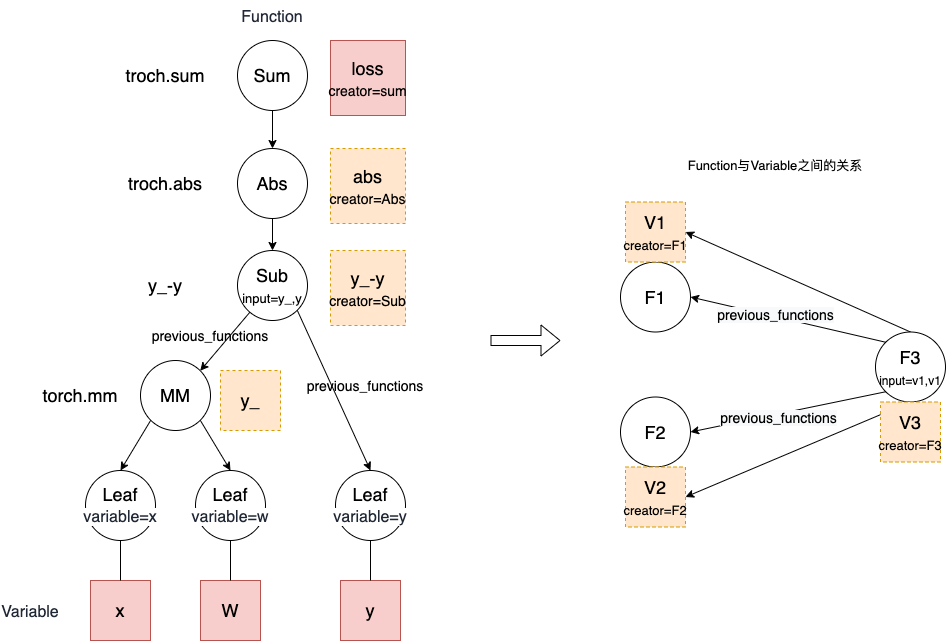y_ - y，对应的方法是 __sub__，开头结尾都是双下划线的方法是 Python 语言中的 magic method，在这里 __sub__ 的作用是重写减法运算符。可以看到减法最终会调用的 Sub 这个类的实例

class Variable(object):
def __sub__(self, other):
return self.sub(other)
def sub(self, other):
if isinstance(other, Variable):
return Sub()(self, other)
else:
return SubConstant(other)(self)
class Sub(Function):
def forward(self, a, b):
return a.sub(b)


Sub 的父类是 Function， Sub()(self, other) 也就是 Function.__call__ ==> Function._do_forward

class Function(object):
def __call__(self, *input):
return self._do_forward(*input)

def _do_forward(self, *input):
unpacked_input = tuple(arg.data for arg in input)
raw_output = self.forward(*unpacked_input)
if not isinstance(raw_output, tuple):
raw_output = (raw_output,)
# 创建 Variable 时 将Function 作为Variable 的creator
output = tuple(Variable(tensor, self) for tensor in raw_output)
# 建立关联关系 ==> 构建 计算图
self.previous_functions = [(arg.creator, id(arg)) for arg in input]
self.output_ids = {id(var): i for i, var in enumerate(output)}
return output
...


### 反向传播

loss.backward
dependencies = Variable._compute_dependencies(variable.creator) ## 从图的终点Function 开始广度优先遍历 所有functions 统计下信息
for (prev_fn, arg_id), d_prev_fn in zip(fn.previous_functions, grad_input):  # 广度优先遍历所有 functions 并执行_do_backward
output_nr = self._free_backward_dependency(dependencies, prev_fn, fn, arg_id)

1. 反向传播传播的啥？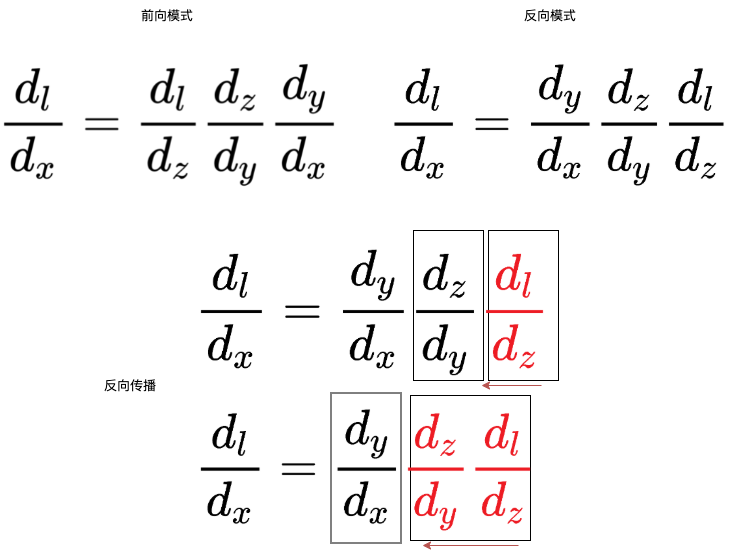2. 以减法 tmp = y_-y 为例，反向 上游 tmp 的梯度 grad_output 通过 Sub.backward 传播 给y_ 的是 grad_output * 1，给y 的是 grad_output * -1，对应到链式法则 就是 $\frac{d_loss}{dy} = \frac{d_loss}{d_tmp} * \frac{d_tmp}{dy}$。
3. 各种Function backward 的逻辑是： $\frac{dl}{df} * \frac{df}{d_input}$ (df 表示当前function)，有几个input 算几个，代码上没按矩阵乘法形式来，直接给了结果。
4. Function.input 可以存储input Variable，估计也可以存储其它状态，比如分段函数 可以在forward 时记录用了哪个分段，backward时就可以 用该分段的导数，这就解释了为什么分段函数也可以反向传播。可以对照 Sub/Mul/Div backward 方法找找感觉。
class Sub(Function):
class Mul(Function):
def forward(self, a, b):
self.input = (a, b)
return a.mul(b)
a, b = self.input
class Div(Function):
def forward(self, a, b):
self.input = (a, b)
return a.div(b)
a, b = self.input


class Leaf(Function):
self.variable = variable
self.output_ids = {id(variable): 0}
self.previous_functions = []
self.backward_hooks = OrderedDict()
def _do_forward(self, *input):
raise NotImplementedError
for hook in self.backward_hooks.values():
return tuple()


### 观察一个运行中的Tensor

Q = {Tensor}
data = {Tensor} tensor(-12.)           # 该张量的数据
device = {device} cpu                      # 存放该张量的设备类型
dtype = {dtype} torch.float32          # 张量的数据类型
grad = {NoneType} None                 # 保存数据data对应的梯度，和数据data的形状一样
next_functions = {tuple: 2}
0 = {tuple: 2} (<MulBackward0 object at 0x000001F9547A5848>, 0)
1 = {tuple: 2} (<PowBackward0 object at 0x000001F9547A53C8>, 0)
__len__ = {int} 2
requires_grad = {bool} True           # 表示该Tensor是否需要求导，如否，在反向传播过程中，该节点所在的子图会被排除在计算过程之外。
is_cuda = {bool} False
is_leaf = {bool} False                 # 记录该张量是否是叶子节点
is_meta = {bool} False
is_mkldnn = {bool} False
is_mlc = {bool} False
is_quantized = {bool} False
is_sparse = {bool} False
is_sparse_csr = {bool} False
is_vulkan = {bool} False
is_xpu = {bool} False
layout = {layout} torch.strided
name = {NoneType} None
names = {tuple: 0} ()
ndim = {int} 0
output_nr = {int} 0
shape = {Size: 0} torch.Size([])


### 各种大杂烩

1. 我们手工编写的forward函数就是pytorch前向运行的动态图。当代码执行到哪一句的时候，网络就运行到哪一步。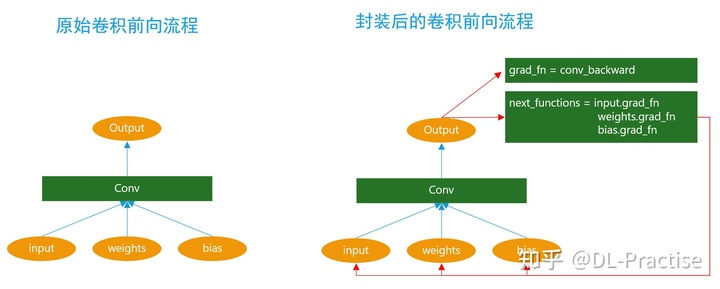5. 每一个前向传播操作的函数都有与之对应的反向传播函数用来计算输入的各个 variable 的梯度，这些函数的函数名通常以Backward结尾。比如 加法对应 AddBackward。 在pytorch v0.1.0 中
class Sub(Function):
def forward(self, a, b):
return a.sub(b)

6. torch.autograd.backward(z) == z.backward() 是等价的，不管正向 反向传播套了多少壳，Pytorch中所有的计算其实都可以回归到Tensor上，矩阵计算。
7. optimizer.step()这个部分, 里面的逻辑也非常简单, 就是刚刚有AccumulateGrad（ AccumulateGrad 会放在 backward 的最后一个 node 拿來做累加 grad） 到的训练参数，就代表需要update它的参数，在optimizer中就会去找那些需要更新。 对应到pytorch v0.1.0 w = Variable(w - 0.1 * w.grad.data,requires_grad=True)

《用python实现深度学习框架》：从计算图中作为结果的节点开始，依次从后向前，每个节点都将结果 对自己的雅克比矩阵 和 自己对父节点的雅克比矩阵 传给父节点，根据链式法则，父节点将这二者想乘就得到 结果对自己的雅克比矩阵。PS： 所以反向传播这块，虽然意思差不多，但各家实现差异还是蛮大的。

## 其它

PyTorch Hub，通过PyTorch Hub，用户可以获得一系列预训练的深度学习模型，主要包括计算机视觉、自然语言处理、生成式模型和音频模型等，这些预训练模型的出现能有效地加快用户开发新型的深度学习模型，方便用户构建基线模型和复现深度学习模型的效果。

PyTorch Lightning工具学习Pytorch Lightning是在Pytorch基础上进行封装的库(可以理解为keras之于tensorflow)，为了让用户能够脱离PyTorch一些繁琐的细节，专注于核心代码的构建，提供了许多实用工具，可以让实验更加高效。 文中以MNIST 为例列出了 使用原生pytorch 和PyTorch Lightning 的代码对比。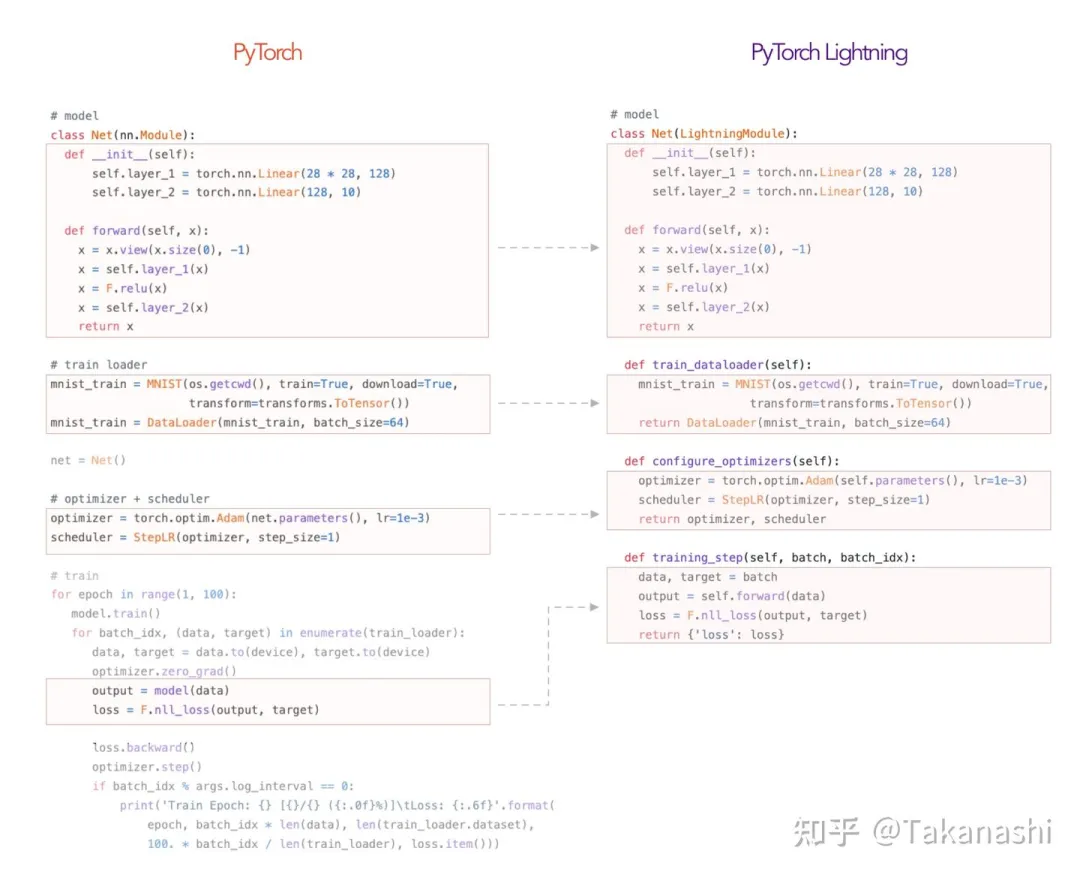PyTorch深度学习技术生态

1. 支持计算图的搭建、前向、反向传播
2. 支持多种不同类型的节点，包括矩阵乘法、加法、数乘、卷积、池化等，还有若干激活函数和损失函数。比如向量操作pytorch 跟numpy 函数命名都几乎一样
3. 提供了一些辅助类和工具函数，例如构造全连接层、卷积层和池化层的函数、各种优化器类、单机和分布式训练器类等，为搭建和训练模型提供便利